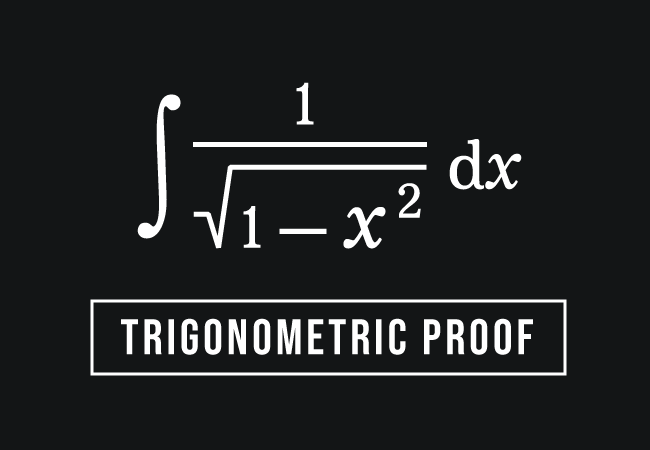# Trigonometric proof of $\displaystyle \int{\dfrac{1}{\sqrt{1-x^2}}\,\,}dx$ RuleThe indefinite integration of one by square root of one minus square of a variable is written in the following mathematical form by considering $x$ as a variable.

$\displaystyle \int{\dfrac{1}{\sqrt{1-x^2}}\,\,}dx$

The integration of the reciprocal (or multiplicative inverse) of this irrational function is actually proved in calculus and it can also be derived in trigonometric method by replacing the variable with a trigonometric function. Now, let’s learn how to derive the integration formula for this function in the trigonometric approach.

### Replacing the variable by a trigonometric function

It is complicated for evaluating the integration of the irrational functions in reciprocal form. However, it can be easily evaluated by substituting a trigonometric function in the variable.

The variable is denoted by $x$ in this case. In trigonometry, sine is a notable function. Hence, let’s assume that the variable $x$ is equal to the sine of angle $y$.

$x \,=\, \sin{y}$

### Differentiate the mathematical equation

The above mathematical equation is defined in two variables $x$ and $y$. Now, find the differentiation of the mathematical equation with respect to $y$.

$\implies$ $\dfrac{d}{dy}{\,(x)}$ $\,=\,$ $\dfrac{d}{dy}{\,(\sin{y})}$

The derivative of $x$ cannot be evaluated with respect to another variable $y$. Hence, the differentiation of the variable $x$ with respect to $y$ is simply written as $dx$ by $dy$. The derivative of sine is cosine as per derivative rule of sine.

$\implies$ $\dfrac{dx}{dy}$ $\,=\,$ $\cos{y}$

$\implies$ $dx$ $\,=\,$ $\cos{y} \times dy$

$\,\,\,\therefore\,\,\,\,\,\,$ $dx$ $\,=\,$ $\cos{y} \, dy$

### Evaluate the Integration of the irrational function

We have taken that $x \,=\, \sin{y}$ and we have also evaluated that $dx$ $\,=\,$ $\cos{y} \, dy$. Now, we can transform the integral function in $x$ in terms of integral function in $y$.

$\implies$ $\displaystyle \int{\dfrac{1}{\sqrt{1-x^2}}\,\,}dx$ $\,=\,$ $\displaystyle \int{\dfrac{1}{\sqrt{1-(\sin{y})^2}}\,\,}(\cos{y}\,dy)$

$\implies$ $\displaystyle \int{\dfrac{1}{\sqrt{1-x^2}}\,\,}dx$ $\,=\,$ $\displaystyle \int{\dfrac{1}{\sqrt{1-\sin^2{y}}}\,\,}(\cos{y}\,dy)$

$\implies$ $\displaystyle \int{\dfrac{1}{\sqrt{1-x^2}}\,\,}dx$ $\,=\,$ $\displaystyle \int{\dfrac{1 \times \cos{y}}{\sqrt{1-\sin^2{y}}}\,\,}dy$

$\implies$ $\displaystyle \int{\dfrac{1}{\sqrt{1-x^2}}\,\,}dx$ $\,=\,$ $\displaystyle \int{\dfrac{\cos{y}}{\sqrt{1-\sin^2{y}}}\,\,}dy$

According to the cos squared identity, the square root of the subtraction of sine squared of angle $y$ from the one is equal to cosine of angle $y$.

$\implies$ $\displaystyle \int{\dfrac{1}{\sqrt{1-x^2}}\,\,}dx$ $\,=\,$ $\displaystyle \int{\dfrac{\cos{y}}{\cos{y}}\,\,}dy$

$\implies$ $\displaystyle \int{\dfrac{1}{\sqrt{1-x^2}}\,\,}dx$ $\,=\,$ $\displaystyle \int{\dfrac{\cancel{\cos{y}}}{\cancel{\cos{y}}}\,\,}dy$

$\implies$ $\displaystyle \int{\dfrac{1}{\sqrt{1-x^2}}\,\,}dx$ $\,=\,$ $\displaystyle \int{1\,\,}dy$

According to the integral of one rule, the integration of one can be evaluated with respect to $y$.

$\implies$ $\displaystyle \int{\dfrac{1}{\sqrt{1-x^2}}\,\,}dx$ $\,=\,$ $y+c$

The integration of the irrational function in reciprocal form is defined in $x$ but its solution is evaluated in $y$. Hence, the value of $y$ should be expressed in terms of $x$.

We have taken that $x \,=\, \sin{y}$. Therefore, $y \,=\, \sin^{-1}{x}$ or $\arcsin{x}$ as per inverse trigonometry.

$\,\,\,\therefore\,\,\,\,\,\,$ $\displaystyle \int{\dfrac{1}{\sqrt{1-x^2}}\,\,}dx$ $\,=\,$ $\sin^{-1}{x}+c$

Therefore, it is evaluated that the indefinite integral of one by square root of one minus $x$ squared with respect to $x$ is equal to the sum of the sine inverse of $x$ and the constant of integration.

Latest Math Topics
Jun 26, 2023
Jun 23, 2023

###### Math Questions

The math problems with solutions to learn how to solve a problem.

Learn solutions

Practice now

###### Math Videos

The math videos tutorials with visual graphics to learn every concept.

Watch now

###### Subscribe us

Get the latest math updates from the Math Doubts by subscribing us.